# Calculate a torque from a pressure

## Homework Statement

HiCalculate the forces F1, F2, F3. F1 is the force on the red wall. F2 is the force on the black center and F3 is the force from springs on the red center. Calculate the torques T1 from F1 and T2 from F2 and give the energy from these torques for and angle of 0.2 radian of the red arm.

The device: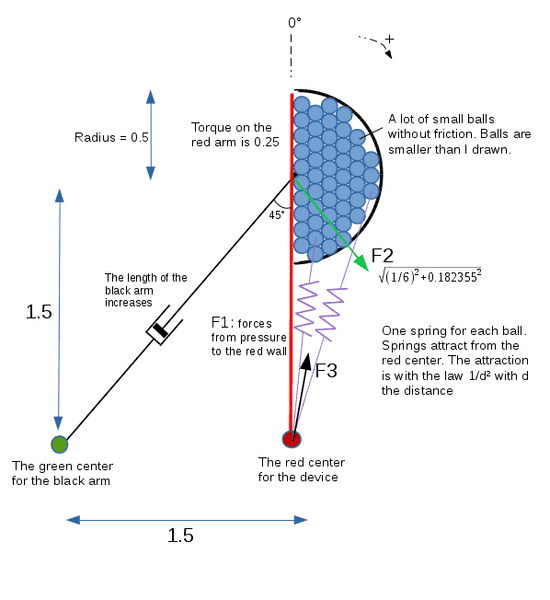The study is in 2 dimensions. The device is composed of a half disk where there are a lot of small balls. The balls have no mass and there is no friction. There are springs that attract balls with the law 1/d² to have the pressure on the walls like gravity can do with water. The springs have no mass. Each spring is attached from the red center to a ball. All the device turns around the red center. The center of the half disk turns too around the red center but it is the black arm that take the black center. Like the centers of rotation are not the same, the black arm must increase its length, I drawn a cylinder for that. The law of attraction of a spring is 1/d² with d the distance from the red center. Note, the springs never lost any potential energy because the balls keep their position in the half disk.

I show the device after 5° of rotation clockwise: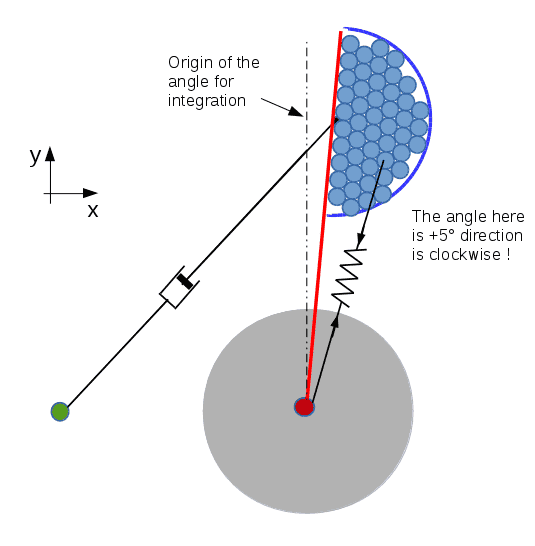## Homework Equations

Torque. Force from pressure.

## The Attempt at a Solution

The forces and the torques:

I found the forces F1, F2 and F3 and I verified the sum is 0 so I think it's correct. I found the torques too but the sum of energy is not 0, so my error come from the forces or the torques: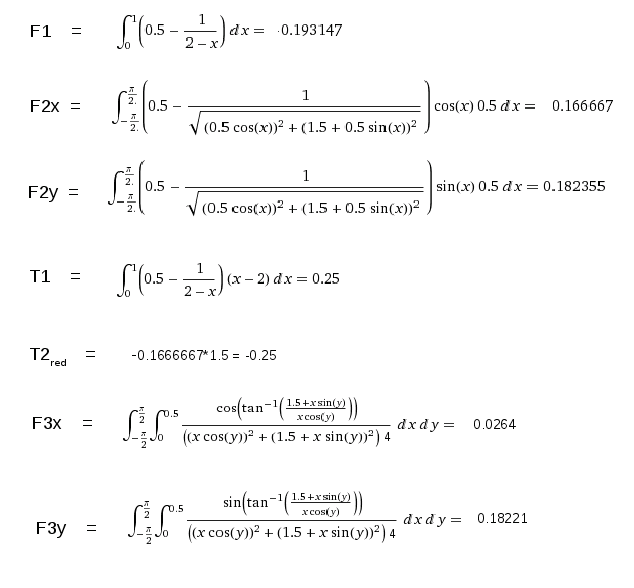The torque T2 I gave above is the torque around the red center not the green center, just for verify the sum of torques is well at 0 around the red center.

I needed to compute F3x and F3y due to a problem of accuracy so maybe I made a mistake, I'm really not sure about F3x and F3y.

Torque T2 and the energy:

I noted :

A: the angle of rotation of the red arm (clockwise), the black arm turns of A/2

##Fx=1/6 ##

##Fy=\int_{-\pi/2}^{\pi/2}( 0.5 - 1/\sqrt( 0.5*\cos(x) + (1.5 + 0.5*\sin(x) ) )*\sin(x)*0.5 dx = 0.182355##

##L=\sqrt( (1.5*\cos(2*x))^2+(1.5+1.5*\sin(2*x))^2)##
I need to have 2x inside trigo functions because when I integrate I do from 0 to A/2

##F=\sqrt( Fx^2 + Fy^2 )##

##B=\arctan( Fx / Fy )##

Torque T2:
##T2= F * L * \sin( pi/4 -x + B )##

Energy recover from black arm:
##\int_0^{A/2}( F * L * \sin( pi/4 -x + B ) dx##

Energy needed to increase the length of the black arm:
##\int_0^{A/2}( F * L ) )* \cos( pi/4 -x + B ) dx##

Energy needed from red arm:
##A*\int_0^1( ( 0.5 – 1/(2-x) ) * (x-2) ) dx##

##\int_0^A/2( F * L * ( \sin( pi/4 - x + B ) - \cos( pi/4 - x + B ) ) dx - A*\int_0^1( ( 0.5 – 1/(2-x) ) * (x-2) ) dx##

But the result is not 0, it is 0.05 - 0.0494171. The difference is small but it is not 0 because the integration take the function sin(x)-cos(x). So, I think I made a mistake about an angle. If you can help me not to find the result just to find the error please ?

Have a good day

Last edited:

CWatters
Homework Helper
Gold Member
This problem might be too difficult for me to help with but nobody else has replied so.....

If the red arm and black/blue half circle are one rigid component then the "springs" cannot cause a torque about the red dot. The springs act towards the red dot so they only act to bend the red arm not rotate it.

My interpretation appears to be confirmed by your second drawing.

It's a theoretical problem, the balls are like molecules of water, very small, no friction. The springs replace the gravity. There are 2 bodies.The red wall is a body, and there is another body: the half circle with its black center. The black arm is fixed on this black center so its length must increase when the device rotates. Sure, like that I supposed, but I forgot to wrote it: An external motor forces the red wall to rotate and follow the half circle because the device must keep its shape (balls inside the half disk). I calculate the energy the motor needs to rotate the red wall and I calculate the energy recover from the black arm. Is it ok for you ?

ps: in reality the device needs gaskets but it's theoretical study

Last edited:
Anybody understand my problem ? The integrals are ok ?

haruspex
Homework Helper
Gold Member
2020 Award
Anybody understand my problem ? The integrals are ok ?
There are many things here I don't get.
What is F2? Is this an external force applied independently of F1 and F3 or a result of them?
What does x represent in your first integral? What does the 0.5 represent?
In your F2 integrals, why do you have 1/d instead of 1/d2, and why do you subtract this from 0.5?

The forces F1 and F2 come from the pressure on the walls, respectively the red wall and the half circle. F2 is the force from the half circle. The fluid gives a pressure to the half circle and I represent the sum of all forces from pressure on the center of the circle. For me, like the force of pressure is perpendicular of the surface, all forces pass through the center of the circle, so I can place F2 on the center.

In the first integral 'x' is the distance. The law of attraction is 1/d² so the pressure at a point is an integral, and the result is 1/2-1/L, for example at the bottom of the half disk I have the pressure 1/2-1/1 = 0.5 (I don't take in account the sign). In my first integral I took the result of an integral, because I need the pressure at a point. '0.5' is 1/2 and 2 is the distance from the red center to the top of the half disk.

In my F2 integral I need to take in account the pressure at a point, so when I integrate 1/d² I have 1/d

The forces F1 and F2 come from the pressure on the walls, respectively the red wall and the half circle. F2 is the force from the half circle. The fluid gives a pressure to the half circle and I represent the sum of all forces from pressure on the center of the circle. For me, like the force of pressure is perpendicular of the surface, all forces pass through the center of the circle, so I can place F2 on the center.

In the first integral 'x' is the distance. The law of attraction is 1/d² so the pressure at a point is an integral, and the result is 1/2-1/L, for example at the bottom of the half disk I have the pressure 1/2-1/1 = 0.5 (I don't take in account the sign). In my first integral I took the result of an integral, because I need the pressure at a point. '0.5' is 1/2 and 2 is the distance from the red center to the top of the half disk.

In my F2 integral I need to take in account the pressure at a point, so when I integrate 1/d² I have 1/d

haruspex
Homework Helper
Gold Member
2020 Award
The forces F1 and F2 come from the pressure on the walls, respectively the red wall and the half circle. F2 is the force from the half circle. The fluid gives a pressure to the half circle and I represent the sum of all forces from pressure on the center of the circle. For me, like the force of pressure is perpendicular of the surface, all forces pass through the center of the circle, so I can place F2 on the center.

In the first integral 'x' is the distance. The law of attraction is 1/d² so the pressure at a point is an integral, and the result is 1/2-1/L, for example at the bottom of the half disk I have the pressure 1/2-1/1 = 0.5 (I don't take in account the sign). In my first integral I took the result of an integral, because I need the pressure at a point. '0.5' is 1/2 and 2 is the distance from the red center to the top of the half disk.

In my F2 integral I need to take in account the pressure at a point, so when I integrate 1/d² I have 1/d
I don't think that answers any of my questions.
Presumably the semicircular wall is rigidly attached to the red wall. If not, it would just slide towards the bottom. I can see that the telescopic rod on the left exerts force F1 on this, but what is exerting force F2 and on what? You seem to have said it is a pressure on the circular wall and comes from the circular wall.
Yes, I understand x is a distance, but what distance?
The force on an individual ball is k/d2, but you cannot add those up by integrating wrt d. Force is a vector, and must be summed according to direction. Integrating wrt d would only make sense for a line of balls lying along the direction of attraction.
Treat the aggregate of balls as a lamina of some constant density. Using polar coordinates centred at the black dot, take an element dr by rdθ, figure out its distance from the red dot, determine the force of attraction on it, resolve that into x and y components, then integrate each of those separately over the semicircle.

Presumably the semicircular wall is rigidly attached to the red wall.
I forgot something important that I added in the message #3, the red wall is not attached to the half circle. There is a motor on the red axis that force the red wall to rotate clockwise and to follow the half disk.

The force on an individual ball is k/d2, but you cannot add those up by integrating wrt d. Force is a vector, and must be summed according to direction. Integrating wrt d would only make sense for a line of balls lying along the direction of attraction.
I think like it was a fluid (it's a theoretical problem: the balls are very small like molecule of water can be and there is not friction), so I used the same law than pressure in a fluid. Pressure of a fluid act on every direction.

but what is exerting force F2 and on what? You seem to have said it is a pressure on the circular wall and comes from the circular wall.
The balls give a pressure to the half circle, each small force from pressure on a small part of the wall pass through the center of the circle. I drawn some forces I know there are a lot of forces, but I can't draw all: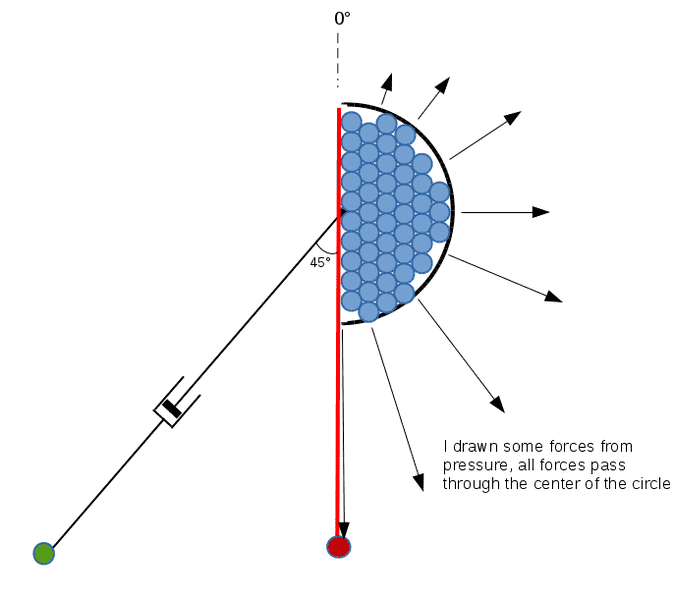haruspex
Homework Helper
Gold Member
2020 Award
I forgot something important that I added in the message #3, the red wall is not attached to the half circle. There is a motor on the red axis that force the red wall to rotate clockwise and to follow the half disk.
I saw that about the force, but took it to refer to F1. But it still does not explain how the semicircle is prevented from sliding towards the red dot.

I saw that about the force, but took it to refer to F1. But it still does not explain how the semicircle is prevented from sliding towards the red dot.

The semicircle has the black axis of rotation (the center of the circle) and this axis is take by the black arm. Look at the image, it's possible to see it under the balls. But I redrawn without the balls and without the red wall: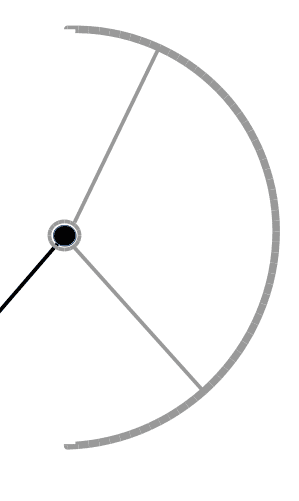I drawn 2 spokes but they are not necessary in the same plan than the balls. I drawn the semicircle with its spokes in gray color (same body). The semicircle can only rotate but it can't because the forces of pressure are always perpendicular of the surface.

haruspex
Homework Helper
Gold Member
2020 Award
The semicircle has the black axis of rotation (the center of the circle) and this axis is take by the black arm. Look at the image, it's possible to see it under the balls. But I redrawn without the balls and without the red wall: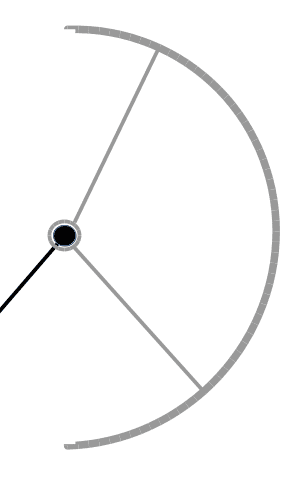I drawn 2 spokes but they are not necessary in the same plan than the balls. I drawn the semicircle with its spokes in gray color (same body). The semicircle can only rotate but it can't because the forces of pressure are always perpendicular of the surface.
Let's see whether I have this right now...
The semicircle is free to rotate about the black dot.
The red arm is joined to, but can rotate about, both the black dot and the red dot.
The telescopic arm is joined to, but can rotate about, the black dot and the green dot.
The ends of the semicircle butt up against the red arm and cannot pass through it.
If all of these are true then the semicircle and the red arm are effectively rigidly joined together, as I surmised in post #7.

I think it is time to stop debating this and proceed with the steps I outlined in post #7.

The semicircle is free to rotate about the black dot.
Correct

The red arm is joined to, but can rotate about, both the black dot and the red dot.
I'm not sure to understand your sentence. The red wall is free to turn around the red axis. But there is a motor that force it to turn clockwise (balls are always at the same position in the half disk). The red axis is fixed to the ground: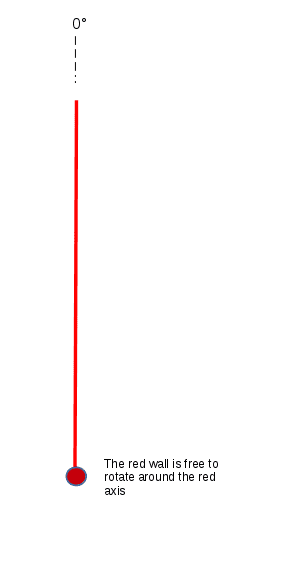The telescopic arm is joined to, but can rotate about, the black dot and the green dot.
The telescopic arm (black arm) is free to rotate around the green axis. The green axis is fixed to the ground.

The ends of the semicircle butt up against the red arm and cannot pass through it.
The ends ? Is it balls ? I don't understand, sorry. If it is balls, correct, balls can't pass through the half disk, there are gaskets.

1/ Red wall: free to rotate around the fixed red axis, a motor force the red wall to rotate clockwise and follow the half disk
2/ The black arm (telescopic) is free to rotate around the fixed green axis, it has the force F2 at end (black dot). The black arm rotates around the green center and increases its length.
3/ Semicircle: free to rotate around the black dot but it don't rotate around it because there is no torque
4/ The trajectory of the black dot is a circle with a radius of 1.5 and the center is the red axis.

Note the black dot is not fixed with the red wall.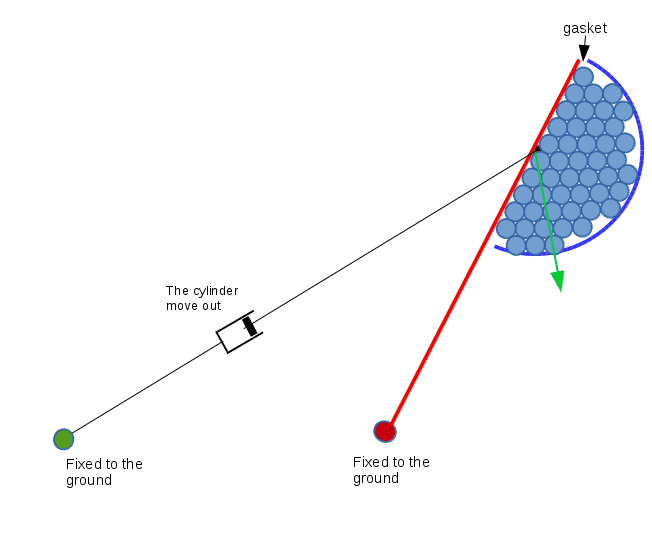Last edited:
haruspex
Homework Helper
Gold Member
2020 Award
If the black dot is not fixed to the red wall then what holds the semicircle against the red wall and stops it slipping down towards the red dot?

If the black dot is not fixed to the red wall then what holds the semicircle against the red wall and stops it slipping down towards the red dot?

The black dot is took by the telescopic black arm. I drawn without the red wall and without the balls: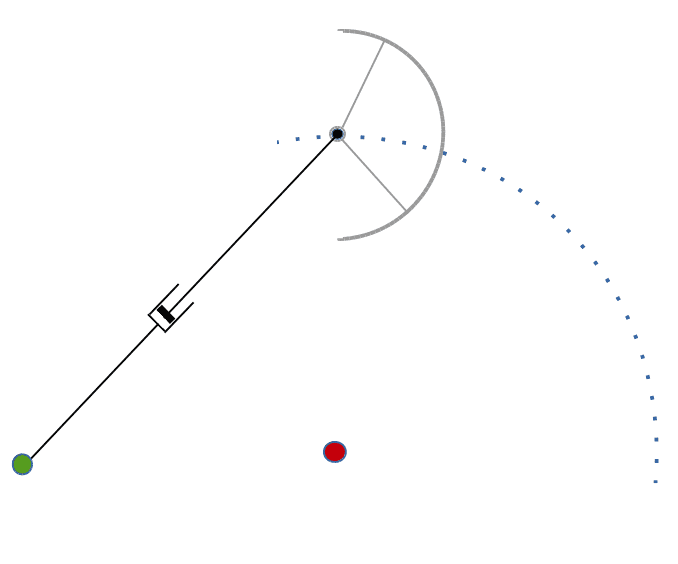The black dot have a circular trajectory (dotted line), radius = 1.5, center = red axis. But this trajectory is controlled by the black arm. The black arm must turn around the green center so it must increase its length. I need to give an energy to increase the length of the black arm and the black arm gives an energy when it rotates. The red wall needs an energy. I don't find the sum at 0.

Dale
Mentor
2020 Award
This is easy to solve using potentials. Your 1/d^2 force is a -1/d potential energy. The system is constrained with a single degree of freedom, and the potential energy is constant along that degree of freedom. Therefore, by conservation of energy there is no torque and no work from the system.

This is easy to solve using potentials. Your 1/d^2 force is a -1/d potential energy. The system is constrained with a single degree of freedom, and the potential energy is constant along that degree of freedom. Therefore, by conservation of energy there is no torque and no work from the system.

I would like to find the error in my integrals. I think it is the work to change the length of the black arm but I don't find the good integral.

The device is not a single body. There is the red wall, it is free to rotate around the fixed red axis. There is the semicircle, it is free to rotate around the black dot. There is the black telescopic arm, it is free to rotate around the fixed green axis.

An external motor gives a clockwise torque to the red wall, so this motor needs an energy because the torque due to the pressure of the balls on the red wall is counterclockwise. The semicircle gives the force F2 to the black dot and like the black arm turns clockwise, the black arm gives an energy. The black arm must increase its length, this needs an energy. I need to find the work with the integrals.

Dale
Mentor
2020 Award
I would like to find the error in my integrals.
The error is using integrals at all. Use conservation of energy and the answer falls out immediately.

Regarding the motor and it's torque. There is no change in potential energy for the system, so the only function of the motor is to change the KE of the system. It's torque can be found simply from that, again without the need of any integrals.

I would like to know if my forces F1, F2 and F3 and my torques T1 and T2 are correct because it is the part of the question. And after, I would like to know if my integrals about the work on the black arm is correct because the solution must use the integrals.

Dale
Mentor
2020 Award
The forces can be found as the gradients of the energy.

I don't know how to use the method of the gradients of the energy. Like I said I need to use the integrals.

Dale
Mentor
2020 Award
Frankly, this doesn't seem like a homework question that an instructor would assign as part of a course. So the idea that you need to use integrals doesn't seem right.

It is a homework, I need to write a program to simulate an object in 2 dimensions with graphical animation, I need to apply in my program integrals (and I can't use library, I need to write all the algorythm). I don't understand why I can't use the integrals, I know the law of attraction, so the pressure, so the force. I have the dimensions of the device so I can calculate with the integrals the forces and the torques. What's wrong ?

Dale
Mentor
2020 Award
You should try a simpler object then. This one is ridiculously hard to do by integrals. It doesn't make sense to even attempt it.

You might try a double pendulum or a pendulum on a cart or something similar.

Maybe you don't understand something in the device. It is not so difficult than that. The balls are small like molecules of water, so I can use the law of pressure with a fluid (no friction). The balls have no mass. Imagine the half disk with water under gravity: I can calculate the forces and the torques, it's easy with integrals. I replace gravity with virtual springs that attract in the red dot the balls, that's all.